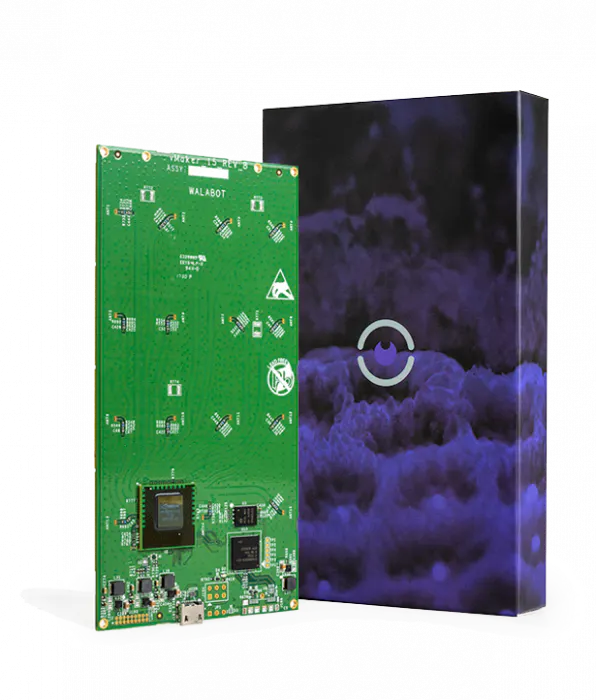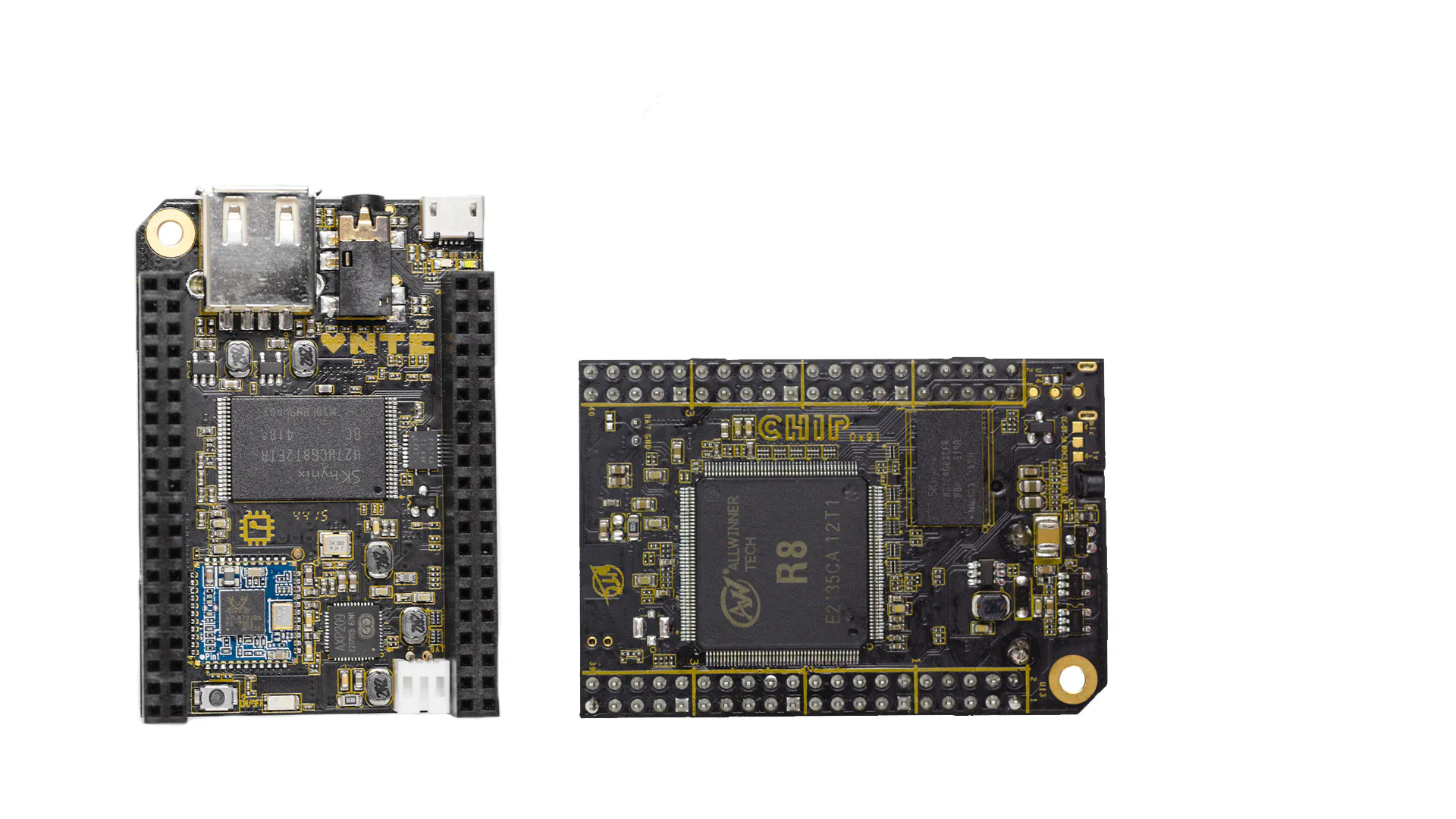# Agent

A device to provide data for Machine Learning.

IntermediateWork in progress2 hours836## Things used in this project

### Hardware componentsWalabot Creator
×1C.H.I.P.
×1

## Code

### An image Classification example

Python
Such an easy script can be used to classify images gathered by walabot.
```import os
import numpy as np
from keras.models import Sequential
from keras.layers import Activation, Dropout, Flatten, Dense
from keras.preprocessing.image import ImageDataGenerator
from keras.layers import Convolution2D, MaxPooling2D, ZeroPadding2D
from keras import optimizers

img_width, img_height = 150, 150

train_data_dir = 'data/train_small'
validation_data_dir = 'data/validation_small'

datagen = ImageDataGenerator(
rescale=1./255,
shear_range=0.2,
zoom_range=0.2,
horizontal_flip=True)

train_generator = datagen.flow_from_directory(
train_data_dir,
target_size=(img_width, img_height),
batch_size=32,
class_mode='binary'
)

validation_generator = datagen.flow_from_directory(
validation_data_dir,
target_size=(img_width, img_height),
batch_size=32,
class_mode='binary'
)

# building the brain
model = Sequential()
model.add(Convolution2D(32, (3, 3), input_shape=(img_width, img_height, 3)))

model.compile(loss='binary_crossentropy',
optimizer='rmsprop',
metrics=['accuracy'])

# training the model
nb_epoch = 30
nb_train_samples = 2048
nb_validation_samples = 832

model.fit_generator(
train_generator,
steps_per_epoch=nb_train_samples/32,
epochs=nb_epoch,
validation_steps=nb_validation_samples/32)

# validate the weights
model.evaluate_generator(validation_generator, nb_validation_samples)
```

## Credits

### Enrico Colautti

1 project • 7 followers
Software developer, DIYer, scuba diver